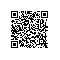# PHP数据库操作：使用ORM

ORM 对象关系映射，O（Object） 对象，在项目中就是实体，更加精确的来说就是数据Model，也可以说持久化类。R（Relation） 关系数据，M (Mapping)映射，将对象映射到关系数据，将关系数据映射到对象的过程。更加直观理解就是，ORM 就是以OOP思想，产生增删改查SQL语句。

## Medoo

### 环境要求

PHP 5.1+, 推荐PHP 5.4+ 且支持PDO.

### 如何安装

Medoo支持Composer安装和直接下载。

composer require catfan/Medoo
composer update

### 开始使用

<?php

//使用Composer安装的这样引入

// 直接下载的这样引入
require_once 'medoo.php';

// 初始化
$db = new medoo([ 'database_type' => 'mysql', 'database_name' => 'test', 'server' => 'localhost', 'username' => 'root', 'password' => '123456', 'charset' => 'utf8', //可选：端口 'port' => 3306, //可选：表前缀 'prefix' => '', // PDO驱动选项 http://www.php.net/manual/en/pdo.setattribute.php 'option' => [ PDO::ATTR_CASE => PDO::CASE_NATURAL ] ]);  如果是SQLite: $database = new medoo([
'database_type' => 'sqlite',
'database_file' => 'my/database/path/database.db'
]);

### CURD

select($table,$columns, $where) //获取所有记录 - table [string] 表名 - columns [string/array] 字段 - where (可选) [array] 查询条件 get($table, $columns,$where) //仅获取一条数据

select($table,$join, $columns,$where)
- table [string] 表名
- join [array] 关联查询，如果没有可以忽略
- columns [string/array] 字段
- where (可选) [array] 查询条件

$user =$db->select('user', '*'); //返回所有数据
$user =$db->get('user', '*'); //返回一条数据
$user =$db->select('user','*', array('name ' => 'joy'));
$user =$db->select('user','name', array('age[>] ' => 20));
$user =$db->select('user',['name','age'], array('age[<=] ' => 20)); 

insert($table,$data)

$db->insert('user', array('name'=> 't3', 'age'=>22)); //返回自增id 注意：如果数据里面包含子数组将会被serialize()序列化, 你可以使用json_encode()作为JSON存储. 更新(Update)： update($table, $data,$where)

$db->update('user', array('name'=> 't5'), array('id'=> 23)); //返回受影响的行数 删除(Delete)： delete($table, $where) 示例： $db->update('user',  array('id'=> 23)); //返回受影响的行数

### 聚合查询

$db->has('user', array('id'=> 23)); //记录是否存在$db->count('user',  array('id[>]'=> 23)); //统计
$db->max('user', 'age', array('gender'=> 1)); //最大值$db->min('user', 'age', array('gender'=> 2)); //最小值
$db->avg('user', 'age', array('gender'=> 2)); //平均值$db->sum('user',  'age', array('gender'=> 2)); //求和

"name" => "foo",
"email" => "bar@abc.com"
]);

$db->delete("account", [ "user_id" => 2312 ]); }catch(Exception$e){
// 返回false就会回滚事务
return false;
}
});

### 使用query

//查询
$data =$db->query("SELECT * FROM user")->fetchAll();
print_r($data); //删除$db->query("DELETE FROM user where name='t5' ");

### 直接使用PDO

Medoo是基于PDO的，所以可以直接调用PDO实例。

$pdo =$db->pdo;

1.PDO::beginTransaction — 启动一个事务
2.PDO::commit — 提交一个事务
3.PDO::__construct — 创建一个表示数据库连接的 PDO 实例
4.PDO::errorCode — 获取跟数据库句柄上一次操作相关的 SQLSTATE
5.PDO::errorInfo — 获取错误信息
6.PDO::exec — 执行一条 SQL 语句,并返回受影响的行数
7.PDO::getAttribute — 取回一个数据库连接的属性
*8.PDO::getAvailableDrivers — 返回一个可用驱动的数组(了解即可)
*9.PDO::inTransaction — 检查是否在一个事务内(了解即可)
10.PDO::lastInsertId — 返回最后插入行的ID或序列值
11.PDO::prepare — 创建SQL的预处理,返回PDOStatement对象
12.PDO::query — 用于执行查询SQL语句,返回PDOStatement对象
13.PDO::quote — 为sql字串添加单引号
14.PDO::rollBack — 回滚一个事务
15.PDO::setAttribute — 设置属性

$stmt =$pdo->query('select * from user limit 2'); //返回一个PDOStatement对象

//$row =$stmt->fetch(); //从结果集中获取下一行，用于while循环
$rows =$stmt->fetchAll(); //获取所有
print_r($rows); pdo事务： $pdo->beginTransaction();//开启事务处理

try{
//PDO预处理以及执行语句...

$pdo->commit();//提交事务 }catch(PDOException$e){
$pdo->rollBack();//事务回滚 //相关错误处理 throw$e;
}

### 使用DEBUG

debug() 打印最终的SQL语句

selectgetinsertupdate等方法前面加上debug()方法可以打印SQL语句，程序不会继续运行：

$user =$db->debug()->select('user', '*');
//SELECT "name","age" FROM "user" WHERE "age" <= 20

error() 返回最后一次操作的出错信息

$db->select('user3', '*'); var_dump($db->error());

log() 返回所有的SQL查询语句，不影响查询正常执行

$db->select('user', '*'); var_dump($db->log());

last_query()log()类似，但仅返回最后一条SQL查询语句，不影响查询正常执行

$db->select('user', '*'); var_dump($db->last_query());

## Eloquent ORM

Eloquent ORM是Laravel框架使用的ORM。Laravel 的 Eloquent ORM 提供了更优雅的ActiveRecord 实现来和数据库的互动。 每个数据库表对应一个模型文件。

1、Guidebook - Medoo
http://medoo.in/api/new/
2、Eloquent ORM笔记 - 飞鸿影~ - 博客园
http://www.cnblogs.com/52fhy/p/5277657.html

(未完待续。。。)使用钉钉扫一扫加入圈子
+ 订阅